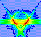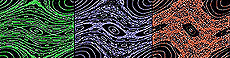Standard Map Main Page Previous | ContinueWhat is a Multi-Dimensional Map? Now that you know that a map is a mathematical transformation, represented by an equation, that can be interated over and over, you can learn what is meant by a multi-dimensional map. The simplest possible case of a multi-dimensional map is a two-dimensional map. The Standard Map is an example of a two-dimensional mapping. For now, however, we will consider a much simpler example.Standard Map Main Page Previous | ContinueWhat is a Multi-Dimensional Map? A two-dimensional map works like this: suppose that to keep track of the position of a point, you need not one but two different measurements. For example, suppose you need to keep track of both the horizontal and vertical positions of a point. You might label the horizontal position as x and the vertical position as y. The variables here arbitrary. You could use any symbols you wanted to, but if you use x and y, people are to know what you are talking about in a straightforward fashion.Standard Map Main Page Previous | ContinueWhat is a Multi-Dimensional Map? As in the case of a one-dimensional map, you need to specify some starting conditions. Suppose that initially, the horizontal position of the point is at 6, and the vertical position is at 24. You would write these starting conditions as: ``` x = 6 (the initial horizontal position) y = 24 (the initial vertical position) ``` Notice that you need two different expressions to specify the initial conditions. ContinueStandard Map Main Page Previous | ContinueWhat is a Multi-Dimensional Map? Since you need two different expressions to specify the initial conditions, it should be no surprise to you that in a two-dimensional map, you need two different rules for how the points will transform. For example, let's suppose that at each iteration of our simple map example, the horizontal position increases by 2, but the vertical position decreases by 4. In mathematical terms, you would write the mapping as ``` x' = x + 2 y' = y - 4 ``` Notice that there are two separate equations, one for each of the two coordinates of the point. As before, the unprimed variables are the values before the map, and the primed variables are the values after the map.Standard Map Main Page Previous | ContinueWhat is a Multi-Dimensional Map? It's important to know that the two mapping equations of our simple example: ``` x' = x + 2 y' = y - 4 ``` are not two different maps, but only a single map. The map is considered to be the set of all the equations that transform the coordinates of the point. In general, you need as many equations in the map as there are coordinates of the point. It should be no surprise that it is quite possible to generalize to three-dimensional maps, and so on, but for our purposes here, two dimensions is enough.Standard Map Main Page Previous | ContinueWhat is a Multi-Dimensional Map? Now that we our initial conditions and our mapping equations, let's see what happens when we iterate the map. Recal that our initial conditions were: ``` x = 6 (the initial horizontal position) y = 24 (the initial vertical position) ``` and our map is: ``` x' = x + 2 y' = y - 4 ``` When the map is iterated with the intial conditions above, we get: ``` x ---> x' y ---> y' 6 8 24 20 (first iteration) 8 10 20 16 (second iteration) 10 12 16 12 (third iteration) 12 14 12 8 (fourth iteration) . . . . (etc.) . . . . . . . . ``` Notice that as before, the "new" primed values after one iteration become the "old" unprimed values for the next iteration. As before, the dots indicate that we could have kept on iterating the map as long as we wanted. In this case, the y-coordinate would have become negative, but there is nothing wrong with that.Standard Map Main Page Previous | ContinueWhat is a Multi-Dimensional Map? So now you know all about two-dimensional maps. But before we conclude this lesson, you should know about a feature of our simple two-dimensional map that wasn't mentioned before. If you look at the equations: ``` x' = x + 2 y' = y - 4 ``` you will notice that to get the new horizontal position x', you only need to know the old horizontal position. The old vertical position y doesn't enter into the mapping equation for x'. Likewise, the old horizontal position x doesn't enter into the equation for y'. In other words, even though the two equations of the map form a set together, they are completely independent of each other in how they act on the coordinates of a point. This type of map is called an uncoupled map.Standard Map Main Page Previous | ContinueWhat is a Multi-Dimensional Map? On the other hand, it is possible to imagine a two-dimensional map with equations like this: ``` x' = x + 2 y' = y - x ``` As before, the horizontal mapping equation depends only on the old horizontal position. But in this case, the vertical mapping equation depends on both the old vertical position and the old horizontal position. The kind of map above is called a coupled map. The two equations of the map are coupled together because in the second equation, both coordinates appear on the right-hand side.Standard Map Main Page Previous | ContinueWhat is a Multi-Dimensional Map? Now let's take a look at what happens when we apply the coupled map to the same pair of initial conditions as before. The initial conditions were: ``` x = 6 y = 24 ``` and the map is: ``` x' = x + 2 y' = y - x ``` When the map is iterated with the above initial conditions, you get: ``` x ---> x' y ---> y' 6 8 24 18 (first iteration) 8 10 18 10 (second iteration) 10 12 10 0 (third iteration) 12 14 0 -12 (fourth iteration) . . . . (etc.) . . . . . . . . ``` Notice how the new y-coordinate is obtained from the old y coordinate and the old x coordinate. This is what happens in any coupled map. By the way, in our example here, only one of the equations is actually coupled. In general, you need only one coupled equation in a map to make it a coupled map. But you could easily imagine a case where all the equations are coupled. ContinueStandard Map Main Page Previous | ContinueWhat is a Multi-Dimensional Map? So now you know all about multi-dimensional maps, uncoupled and coupled. To a physicist, uncoupled maps are pretty uninteresting. Coupled maps are where all the action is, since they are the ones that show interesting dynamical processes. But it takes more than a coupled map to make chaos. Continue